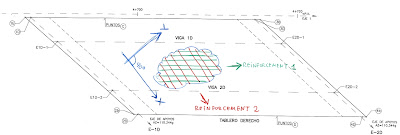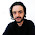# Blog of eng. Xosé Carreira (since 2007)

Structures, water, computers, languages and people (not necessarily in this order)

### The skew (or non-orthogonal) reinforcement problem

Reinforcement that is not perperdicular or that is arranged in more than two directions occurs frequently in the design of slabs for skew bridges. In such cases, reinforcement can be calculated with an equivalent orthogonal reinforcement.Given n arbitrarily oriented groups of paralel bars r1, r2, ...., rn, an equivalent reinforcement (ru, rv, ruv) for an auxiliar set UV of perpendicular axes are obtained by projection:

ru = r1*(cos(a1))^2 + ... + rn*(cos(an))^2
rv = r1*(sin(a1))^2 + ... + rn*(sin(an))^2
ruv = r1*cos(a1)sin(a1) + ... + rn*cos(an)sin(an)

These values are transformed into the principal directions of reinforcement XY (that are obviously orthogonal) like this:

rx = ru*(cos(b))^2+rv*(sin(b))^2+ruv*sin(2*b)
ry = rv*(sin(b))^2+rv*(cos(b))^2-ruv*sin(2*b)

where

b= 0.5*arctan(2*ruv/(ru-rv))

Now, the axial and flexural resistance should be confronted with the forces and moments given in the XY system of coordinates. Changing the local axis of the shell elements we obtain Nx, Ny, Nxy and Mx, My, Mxy.The Wood and Armer rule (or any other similar) can be applied now.

rx should resist Nx' and Mx'
ry should resist Ny' and My'

For a symmetric ultimate reinforcement with Nxy and Mxy not extremely large, Wood and Armer rule becomes.

Nx' = Nx +/- |Nxy|
Ny' = Ny +/- |Nxy|
Mx' = Mx + sign(Mx)*|Mxy|
My' = My + sign(My)*|Mxy|

1.2.